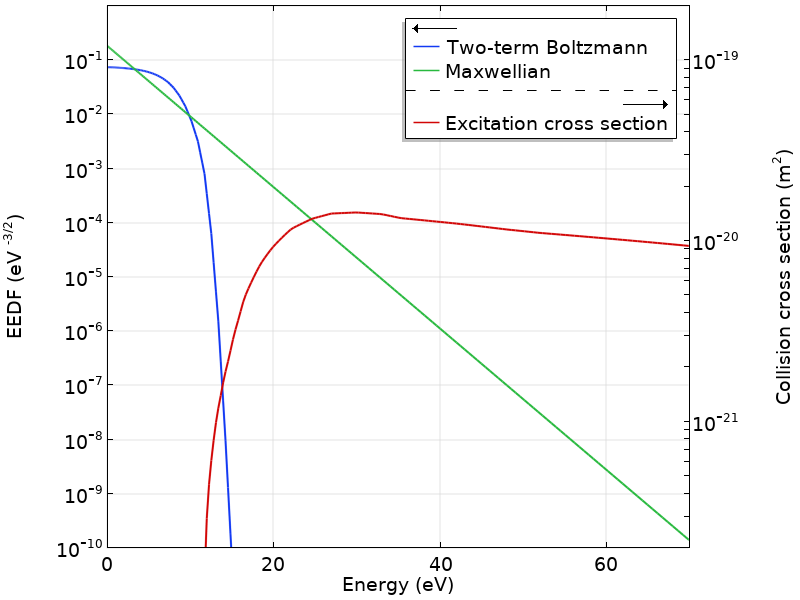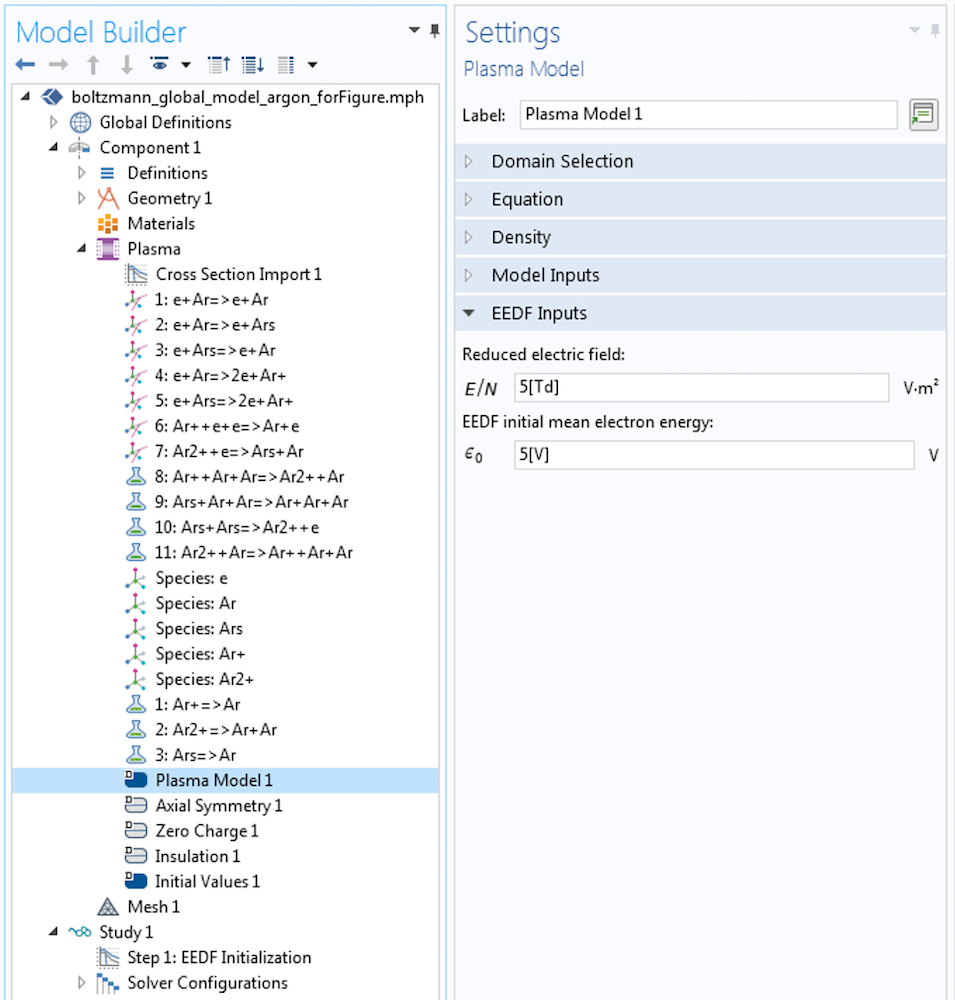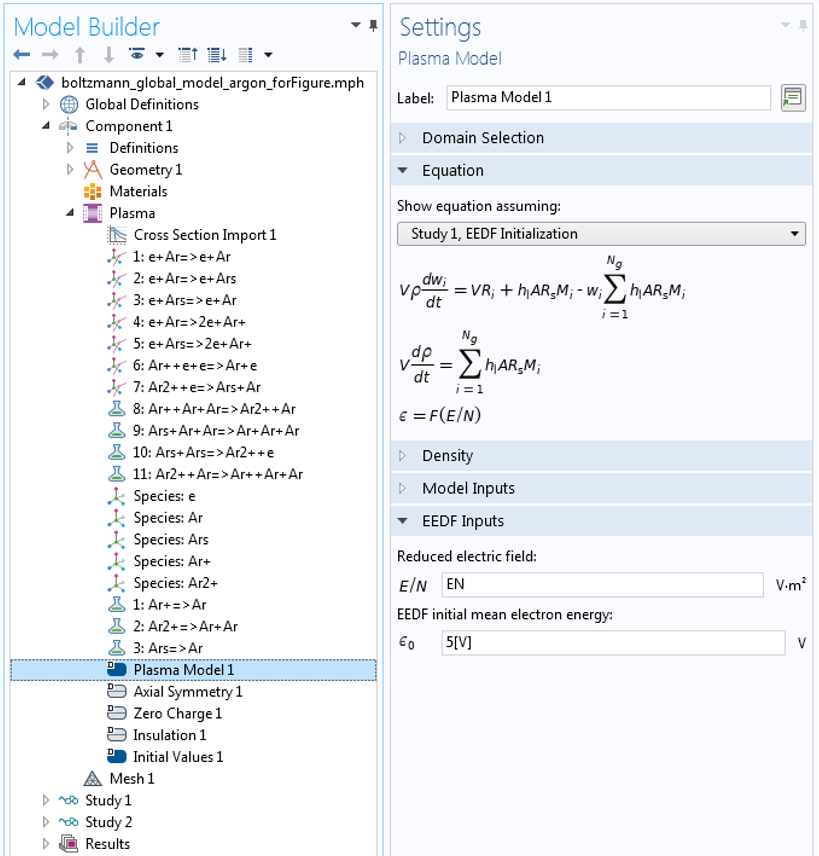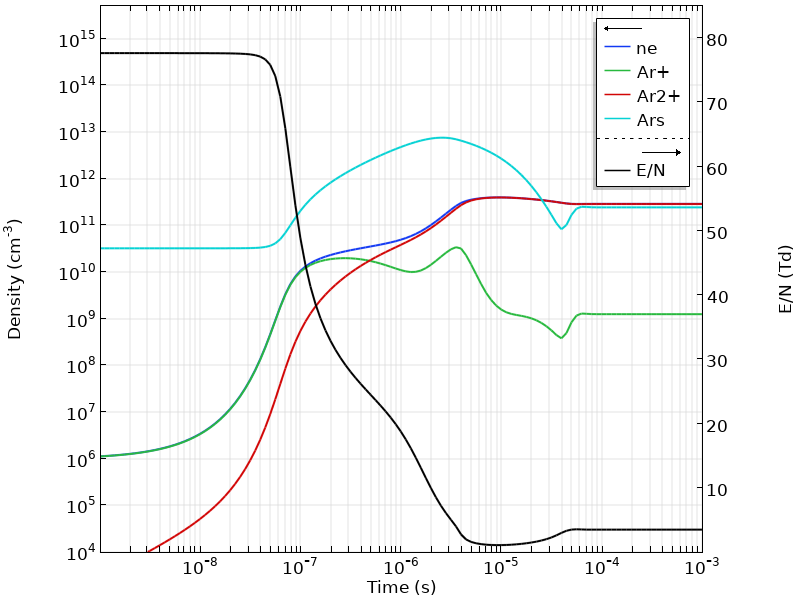# 使用 COMSOL® 对非麦克斯韦放电进行全局建模

2018年 11月 19日

### 建立非麦克斯韦放电的全局模型

V \rho \frac{d w_k}{dt}= VR_k+\sum_{l}h_l A_l R_{surf,k,l}M_k

n_e=\sum_{k=1}^N Z_k n_k

e\frac{d n_{\varepsilon}}{dt}=R_{\varepsilon}\frac{P_{abs}}{V}\sum_l \sum_{ions} e h_l \frac{A_l}{V}R_{surf,k,l} N_A \left( \varepsilon_e + \varepsilon_i \right)

### 玻尔兹曼方程和二项近似

\frac{\partial f}{\partial t}+\textbf{v} \cdot \nabla f-\frac{e}{m}\textbf{E} \cdot \nabla_v f=C[f]

f\left( v,cos \theta,z ,t \right) = f_0 \left( v, z ,t \right) + f_1\left( v,z ,t \right)cos \theta

f_{0,1} \left( \varepsilon, z, t \right) = \frac{1}{2 \pi \gamma^3}F_{0,1} \left( \varepsilon \right) n\left( z, t\right)

\int_0^\infty \varepsilon^{1/2} F_0 d\varepsilon =1

\frac{\partial }{\partial \varepsilon} \left( W F_0-D \frac{\partial F_0 }{\partial \varepsilon}
\right) = S

（有关更多详细信息，请参见参考文献1。）

\int_0^\infty \varepsilon^{3/2} F_0 d\varepsilon =\overline{\varepsilon}

k_k=\gamma \int_0^\infty \varepsilon \sigma_k \left( \varepsilon \right) F_0\left( \varepsilon \right) d\varepsilon### 在二项近似中将全局模型与玻尔兹曼方程耦合

1. 创建使用解析EEDF的全局模型
2. 使用 EEDF 初始化 研究仅求解 EEDF
3. 求解完全耦合的问题

#### 创建 EEDF 初始化研究#### 耦合全局模型方程和玻尔兹曼方程

\int_0^\infty \varepsilon^{3/2} F_0 \left( E/N,n_k/N,n_e/N,\dotsc\right) d\varepsilon-\overline{\varepsilon}=0.

#### 示例：由直流电压源维持的等离子体

V_p=V_{dc}-RI_p

I_p=e A n_e \left( \mu N\right) \left(E/N\right)

\frac{E}{N}=\frac{V_{dc}}{dN+e R A n_e \left( \mu N \right)}P_{abs}=V_p I_pEEDF 的时间演变如下图所示。最初，由于有必要促进等离子体击穿，因此EEDF高于 15eV。在等离子体形成之后，由于电场的降低，电子群冷却下来，EEDF 形成了一个斜率更陡的尾部。随着时间的流逝，并且随着氩激发态密度的增加，EEDF中超弹性反应的影响变得明显，高能量端出现了凸起。请注意，此动画中的时间变化以对数刻度显示。

### 参考文献

1. J.M. Hagelaar and L.C. Pitchford, “Solving the Boltzmann equation to obtain electron transport coefficients and rate coefficients for fluid models,” Plasma Sources Science and Technology, vol. 14, pp. 722–733, 2005.
2. Pancheshnyi, B. Eismann, G. Hagelaar, and L. Pitchford, “ZDPlasKin: A New Tool for Plasmachemical Simulations”, The Eleventh International Symposium on High Pressure, Low Temperature Plasma Chemistry, 2008.